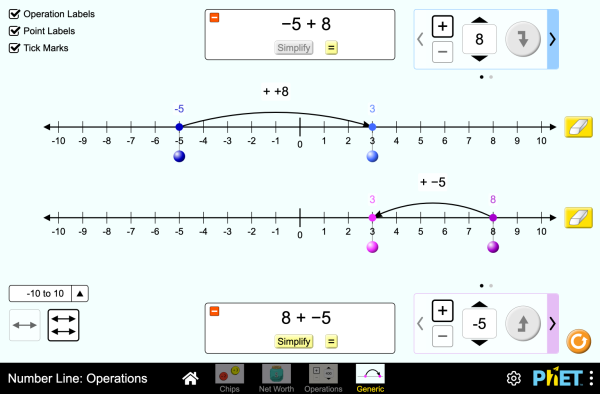# Бројевна права: ОперацијеПреузимање (Download)Уградња (Embed) затвори Угради (Ембедуј) извршну копију ове симулације користи овај HTML за уградњу (embed) извршне копије ове симулације. Можеш мењати домензије приказа (ширину и висину екрана) мењајући "width" и "height" атрибуте у HTML. Угради слику. Кликом на њу покретаће се симулација.
Кликни и започни
Користи овај HTML код да би се појавио екран са натписом "Кликни и започни" ("Click to Run"). Operations Number Line Integers PhET је подржан од:и наставника као што сте Ви!

• Operations
• Number Line
• Integers

### Опис

Explore operations with integers using an authentic context of net worth, then generalize with a number line. Experiment with adding and subtracting both positives and negatives, and make predictions about whether the total will be positive or negative.

### Примери образовних циљева

• Represent addition and subtraction of integers on a horizontal number line
• Reason about addition and subtraction of integers in terms of number-locations
• Use logical necessity to reason that addition has the opposite effect as subtraction or that adding (or subtracting) a negative integer has the opposite effect as adding (or subtracting) a positive integer.
• Recognize and generate equivalence classes of integer sums and differences
• Show that a number and its additive inverse (opposite) have a sum of 0
• Using a net worth context, describe situations that have a positive sum or difference, negative sum or difference, and zero sum or difference
• View adding a negative as equivalent to subtracting a positive, and explain why this relationship makes sense in contexts such as net worth.
• View subtracting a negative as equivalent to adding a positive, and explain why this relationship makes sense in contexts such as net worth.
• Apply a number line model for addition and subtraction to new contexts.

### Усклађивање стандарда

#### Заједничко језгро - математика

7.NS.A.1
Apply and extend previous understandings of addition and subtraction to add and subtract rational numbers; represent addition and subtraction on a horizontal or vertical number line diagram.
7.NS.A.1a
Describe situations in which opposite quantities combine to make 0. For example, a hydrogen atom has 0 charge because its two constituents are oppositely charged.
7.NS.A.1b
Understand p + q as the number located a distance |q| from p, in the positive or negative direction depending on whether q is positive or negative. Show that a number and its opposite have a sum of 0 (are additive inverses). Interpret sums of rational numbers by describing real-world contexts.
7.NS.A.1c
Understand subtraction of rational numbers as adding the additive inverse, p - q = p + (-q). Show that the distance between two rational numbers on the number line is the absolute value of their difference, and apply this principle in real-world contexts.
7.NS.A.1d
Apply properties of operations as strategies to add and subtract rational numbers.
Верзија 1.0.1

### Савети за наставникеПреглед контроле симулација, поједностављења модела и увид у размишљање студената ( PDF ).

### Активности наставника

Integer Addition and Subtraction Assessment Gillian Walker HS (ВШ)
MS (СШ)
Remote
Лаб
ДЗ
Математика
Holandski Све NederlandsGetallijn: Bewerkingen
Баскијски Све EuskaraZenbaki-lerroa: eragiketak
Бошњачки Све BosanskiBrojevna prava: Operacije
Бразилски Португалски Све português (Brasil)Reta Numérica: Operações
Вијетнамски Све Tiếng ViệtĐường thẳng số: Các phép toán
Грчки Све ΕλληνικάΑριθμογραμμή: πράξεις
Дански Све DanskTallinje: Beregninger
Енглески Све EnglishNumber Line: Operations
Италијански Све italianoRetta dei numeri: operazioni
Кинески - Традиционални Све 中文 (台灣)Number Line: Operations_數線：數線的運算
Летонски Све LatviešuSkaitļu ass: Darbības
Мађарски Све magyarSzámegyenes - műveletek
Маорски Све MaoriRārangi Tau: Paheko
Немачки Све DeutschZahlenstrahl: Operationen
Пољски Све polskiOś liczbowa: działania
Словачки Све SlovenčinaČíselná os: Operácie
Турски Све TürkçeSayı Doğrusu: İşlemler
Француски Све françaisDroite numérique: opérations
Хрватски Све hrvatskiBrojevna crta: Računske operacije
Шпански Све españolRecta Numérica: OperacionesШпански (Перу) Све español (Perú)Recta numérica: Operaciones

HTML5 sims can run on iPads and Chromebooks, as well as PC, Mac, and Linux systems on modern web browsers. If you are experiencing issues using an HTML5 sim on a supported platform, please disable any browser extensions.

iOS 12+ Safari

Android:
Not officially supported. If you are using the HTML5 sims on Android, we recommend using the latest version of Google Chrome.

Chromebook:
The HTML5 and Flash PhET sims are supported on all Chromebooks.
Chromebook compatible sims

Windows Systems: